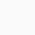Course

# Introduction to Data Science and Basic Statistics for Business

👤 Join WhatsApp Learners
Deal Score0

In this course, you will acquire statistical methods for decision-making in business, as well as technological tools to develop quantitative skills.

### Join Email Learners

Follow the guide on landing page

Areas such as “big data” require very clear knowledge of statistics and business, and technology provides us with various applications that require solid training in statistics for proper use and interpretation.

This course allows you to develop the skills of a decision-maker leader based on the following competencies:

• analysis of statistical elements of information
• concepts and statistical foundations for the application of the area of ​​data science

Through descriptive statistics, the study and analysis of discrete and continuous probability distributions, as well as the estimation by intervals for the mean and the proportion, it is how you will be able to develop both skills.

Through this course, participants will be able to:

● Apply basic statistical methods to the business environment

● Use technological tools to develop quantitative skills

● Interpret the results of statistical methods applied to businesses

Topic 1: Descriptive statistics

1.1 Types of data; Measurement scales; Organization of qualitative and quantitative data; Graphic representation;

1.2 Measures of central tendency; Position measurements; Measures of dispersion; Descriptive statistics on your laptop

Topic 2: Discrete Probability Distributions

2.1 Discrete random variable; Density and cumulative probability functions; Mean and variance of a discrete random variable; Fundamental properties;

2.2 Poisson distribution ; Binomial Distribution

Topic 3: Continuous Probability Distributions

3.1 Continuous random variable; Density and cumulative probability functions; Mean and variance of a continuous random variable; Fundamental properties;

3.2 Uniform Distribution; Normal distribution

Topic 4: Interval estimation for the mean and proportion

4.1 Confidence intervals calculation; Calculation of sample size required for a given estimation error;

4.2 Student’s t distribution.

Introduction to Data Science and Basic Statistics for Business
0